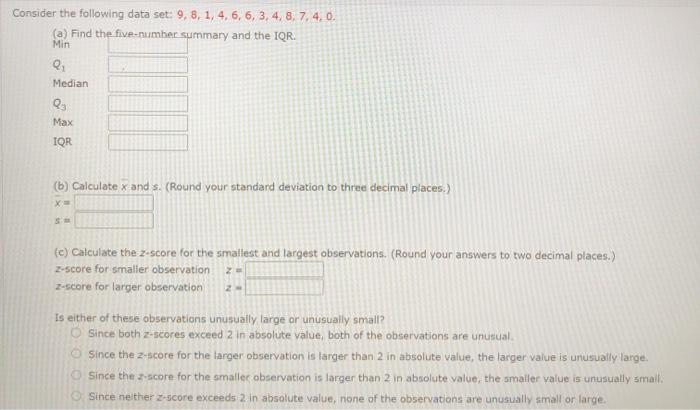# Question Solved1 AnswerConsider the following data set: 9, 8, 1, 4, 6, 6, 3, 4, 8, 7, 4, 0. (a) Find the five-number summary and the IQR. Min Q Median Q Max IQR (b) Calculate x and s. (Round your standard deviation to three decimal places.) X (c) Calculate the z-score for the smallest and largest observations. (Round your answers to two decimal places.) Z-score for smaller observation Z-score for larger observation Is either of these observations unusually large or unusually small? Since both Z-scores exceed 2 in absolute value, both of the observations are unusual Since the 2-score for the larger observation is targer than 2 in absolute value, the larger value is unusually large. Since the score for the smaller observation is larger than 2 in absolute value, the smaller value is unusually small. Since neither z-score exceeds 2 in absolute value, none of the observations are unusually small or large.KKCRRD The Asker · Probability and StatisticsTranscribed Image Text: Consider the following data set: 9, 8, 1, 4, 6, 6, 3, 4, 8, 7, 4, 0. (a) Find the five-number summary and the IQR. Min Q Median Q Max IQR (b) Calculate x and s. (Round your standard deviation to three decimal places.) X (c) Calculate the z-score for the smallest and largest observations. (Round your answers to two decimal places.) Z-score for smaller observation Z-score for larger observation Is either of these observations unusually large or unusually small? Since both Z-scores exceed 2 in absolute value, both of the observations are unusual Since the 2-score for the larger observation is targer than 2 in absolute value, the larger value is unusually large. Since the score for the smaller observation is larger than 2 in absolute value, the smaller value is unusually small. Since neither z-score exceeds 2 in absolute value, none of the observations are unusually small or large.
More
Transcribed Image Text: Consider the following data set: 9, 8, 1, 4, 6, 6, 3, 4, 8, 7, 4, 0. (a) Find the five-number summary and the IQR. Min Q Median Q Max IQR (b) Calculate x and s. (Round your standard deviation to three decimal places.) X (c) Calculate the z-score for the smallest and largest observations. (Round your answers to two decimal places.) Z-score for smaller observation Z-score for larger observation Is either of these observations unusually large or unusually small? Since both Z-scores exceed 2 in absolute value, both of the observations are unusual Since the 2-score for the larger observation is targer than 2 in absolute value, the larger value is unusually large. Since the score for the smaller observation is larger than 2 in absolute value, the smaller value is unusually small. Since neither z-score exceeds 2 in absolute value, none of the observations are unusually small or large.